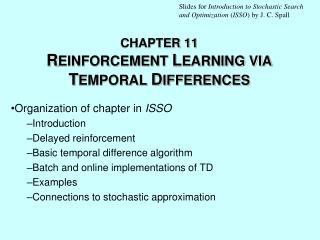Download PresentationCHAPTER 11 R EINFORCEMENT L EARNING VIA T EMPORAL D IFFERENCES

# CHAPTER 11 R EINFORCEMENT L EARNING VIA T EMPORAL D IFFERENCES - PowerPoint PPT PresentationDownload Presentation## CHAPTER 11 R EINFORCEMENT L EARNING VIA T EMPORAL D IFFERENCES

- - - - - - - - - - - - - - - - - - - - - - - - - - - E N D - - - - - - - - - - - - - - - - - - - - - - - - - - -
##### Presentation Transcript

1. Slides for Introduction to Stochastic Search and Optimization (ISSO)by J. C. Spall CHAPTER 11REINFORCEMENT LEARNING VIA TEMPORAL DIFFERENCES Organization of chapter in ISSO Introduction Delayed reinforcement Basic temporal difference algorithm Batch and online implementations of TD Examples Connections to stochastic approximation

2. Reinforcement Learning • Reinforcement learning is important class of methods in computer science, AI, engineering, etc. • Based on common-sense idea that good results are reinforced while bad results provide negative reinforcement • Delayed reinforcement only provides output after several intermediate “actions” • Want to create model for predicting state of system • Model depends on  • “Training” or “learning” (estimating ) not based on methods such as stochastic gradient (supervised learning) because of delay in response • Need learning method to cope with delayed response

3. Schematic of Delayed Reinforcement Process • Suppose time moves left to right in diagram below • Z represents some system output at a future time • represent some intermediate predictions of Z

4. Temporal Difference (TD) Learning • Focus is delayed reinforcement problem • Prediction function has form ht(,xt), where  are parameters and xt is input • Need to estimate  from sequence of inputs and outputs {x0, x1, ..., xn; Z} • TD learning is method for using in training rather than only inputs and outputs • Implies that some forms of TD allow for updating of  value before observing Z • TD exploits prior information embedded in predictions to modify  • Basic form of TD for updating  is where () is increment to be determined

5. Exercise 11.4 in ISSO: Conceptual Example of Benefits of TD. Circles denote game states. Loss 90% Bad Novel 10% Win Game outcome

6. Batch Version of TD Learning

7. Tleft S1 S2 Tright S3 S5 S4 Random-Walk Model(Example 11.3 in ISSO) • All walks begin in state S3 • Each step involves 50–50 chance of moving left or right until terminal state Tleftor Tright is reached • Use TD to estimate probabilities of reaching Tright from any of states S1, S2, S3, S4, or S5 Start